Download Presentation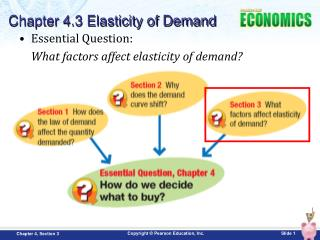Essential Question: What factors affect elasticity of demand?

# Essential Question: What factors affect elasticity of demand? - PowerPoint PPT Presentation

Chapter 4.3 Elasticity of Demand. Essential Question: What factors affect elasticity of demand?. Objectives. Explain what is and how to calculate elasticity of demand. Identify factors that effect elasticity. What is Elasticity of Demand?.I am the owner, or an agent authorized to act on behalf of the owner, of the copyrighted work described.
Download Presentation## Essential Question: What factors affect elasticity of demand?

An Image/Link below is provided (as is) to download presentation

Download Policy: Content on the Website is provided to you AS IS for your information and personal use and may not be sold / licensed / shared on other websites without getting consent from its author.While downloading, if for some reason you are not able to download a presentation, the publisher may have deleted the file from their server.

- - - - - - - - - - - - - - - - - - - - - - - - - - E N D - - - - - - - - - - - - - - - - - - - - - - - - - -
Presentation Transcript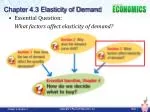Chapter 4.3 Elasticity of Demand

• Essential Question:

What factors affect elasticity of demand?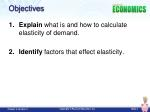Objectives

Explain what is and how to calculate elasticity of demand.

Identify factors that effect elasticity.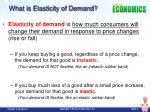What is Elasticity of Demand?
• Elasticity of demand is how much consumers will change their demand in response to price changes (rise or fall)
• If you keep buying a good, regardless of a price change, the demand for that good is inelastic.

(Your demand IS NOT flexible, like an inelastic rubber band)

• If you buy much less of a good after a small price increase, your demand for that good is elastic.

(Your demand IS flexible, like an elastic rubber band)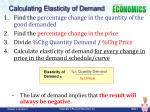Calculating Elasticity of Demand
• Find the percentage change in the quantity of the good demanded
• Find the percentage change in the price
• Divide %Chg Quantity Demand / %Chg Price
• Calculate elasticity of demand for every change in price in the demand schedule/curve
• The law of demand implies that the result will always be negative.

%∆ Quantity Demand

Elasticity of Demand =

%∆ Price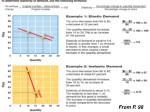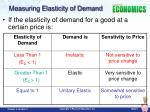Measuring Elasticity of Demand
• If the elasticity of demand for a good at a certain price is: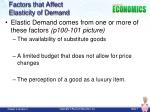Factors that Affect Elasticity of Demand
• Elastic Demand comes from one or more of these factors (p100-101 picture)
• The availability of substitute goods
• A limited budget that does not allow for price changes
• The perception of a good as a luxury item.Factors that Affect Elasticity of Demand

• Availability of Substitutes
• If there are a few substitutes for a good, then even when its price rises greatly, you might still buy it.
• Few substitutes can make demand inelastic
• Many substitute goods can make demand elastic.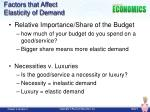Factors that Affect Elasticity of Demand

• Relative Importance/Share of the Budget
• how much of your budget do you spend on a good/service?
• Bigger share means more elastic demand
• Necessities v. Luxuries
• Is the good/service a necessity or luxury?
• Necessity = inelastic demand
• Luxury = elastic demand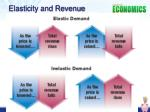Key Terms
• elasticity of demand: a measure of how consumers respond to price changes
• inelastic: describes demand that is not very sensitive to price changes
• elastic: describes demand that is very sensitive to a change in price
• unitary elastic: describes demand whose elasticity is exactly equal to 1
• total revenue: the total amount of money a company receives by selling goods or services